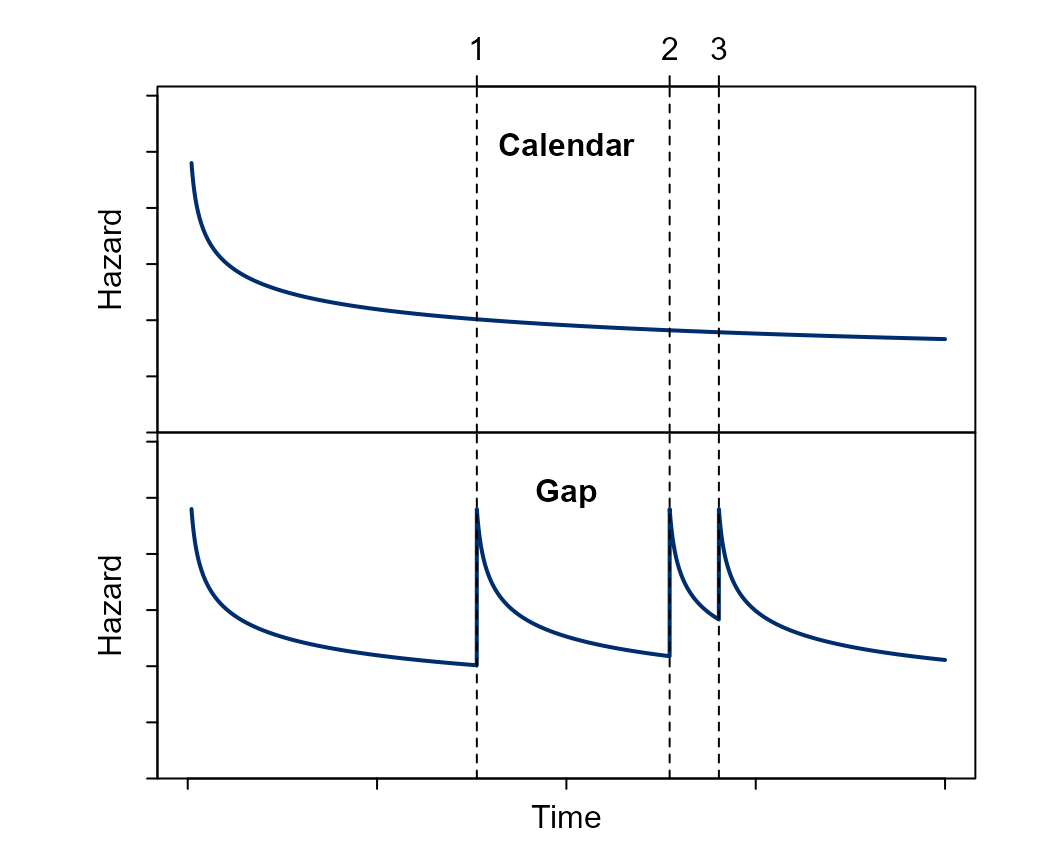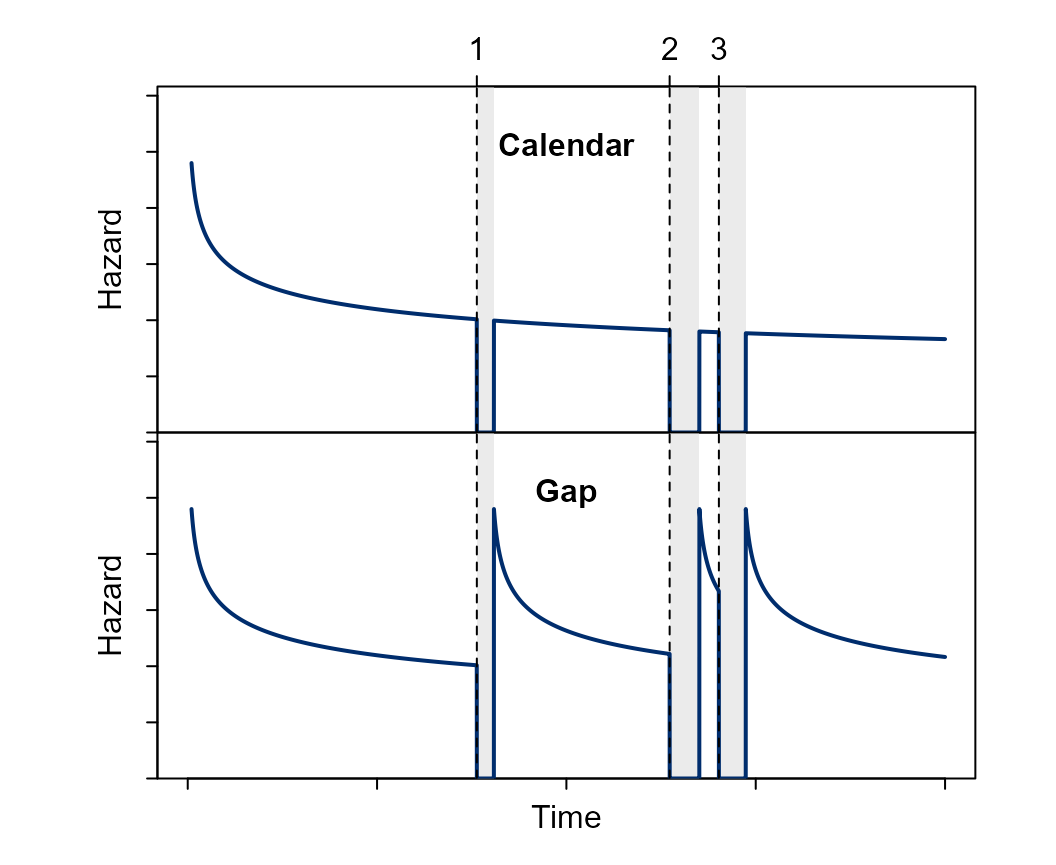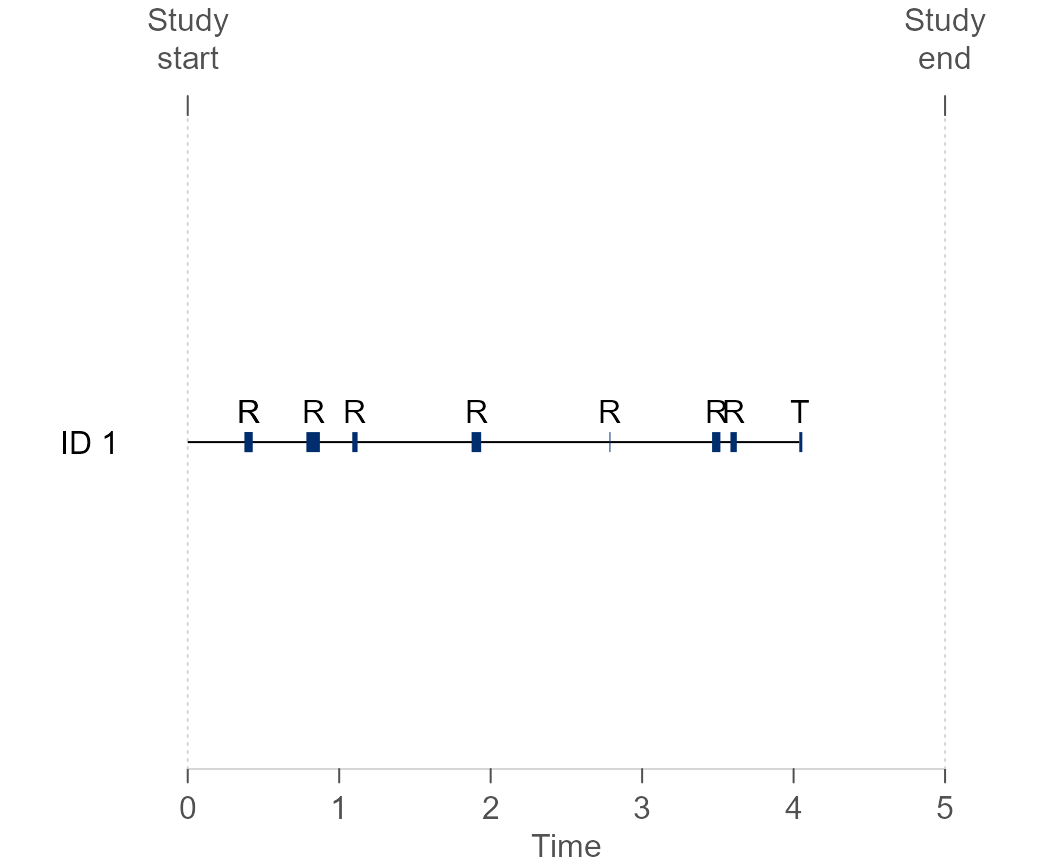## Recurrent events

### Introduction

JMbayes2 provides also the capability to fit joint models with a recurrent event process possibly combined with a terminating event. Recurrent events are correlated events that may occur more than once over the follow-up period for a given subject. Our current implementation allows for multiple longitudinal markers with different distributions, and various functional forms to link these markers with the risk of recurrent and terminal events. Furthermore, it enables the risk intervals to be defined in terms of the gap or calendar timescales. The two timescales use a different zero-time reference. The calendar uses the study entry, while the gap uses the end of the previous event (Figure 1). Gap assumes a renewal after each event and resets the time to zero.Figure 1 Visual representation of an hazard function under the gap or calendar timescale. During the folow-up the subject experienced three events.

The model also accommodates discontinuous risk intervals, i.e., periods in which the subject is not at risk of experiencing the recurring event (Figure 2). For example, while a patient is in the hospital, they are not at risk of being hospitalized again.Figure 2 Visual representation of an hazard function under the gap or calendar timescale, while accounting for non-risk periods (gray areas). During the folow-up the subject experienced three events.

A joint model with $$p$$ normally distributed longitudinal markers, a terminal event process, and a recurrent event process can be described as follows: $\small{\require{color} \begin{cases} y_{1_i}(t)= \colorbox{#79FCFD80}{ x_{1_i}(t)^\top\beta_1 + z_{1_i}(t)^\top b_{1_i}} + \varepsilon_1(t) = \colorbox{#79FCFD80}{ \eta_{1_i}(t)} + \varepsilon_1(t) & \text{Longitudinal marker 1}\\ \vdots \\ y_{p_i}(t)= \colorbox{#FCFF5580}{ x_{p_i}(t)^\top\beta_p + z_{p_i}(t)^\top b_{p_i}} + \varepsilon_p(t) = \colorbox{#FCFF5580}{ \eta_{p_i}(t)} + \varepsilon_p(t) & \text{Longitudinal marker p}\\ h_{T_i}(t)= h_{T_0}(t)\exp\left\{ w_{T_i}(t)^\top \gamma_T + \colorbox{#79FCFD80}{\mathcal{f}_{T_1}\left\{\eta_{1_i}(t)\right\}} \alpha_{T_1} + \dots + \colorbox{#FCFF5580}{\mathcal{f}_{T_p}\left\{\eta_{p_i}(t)\right\}} \alpha_{T_p} + \colorbox{#5FFFAB80}{b_{F_i}} \alpha_{F} \right\} & \text{Terminal event}\\ h_{R_i}(t)= h_{R_0}(t)\exp\left\{ w_{R_i}(t)^\top \gamma_R + \colorbox{#79FCFD80}{\mathcal{f}_{R_1}\left\{\eta_{2_i}(t)\right\}} \alpha_{R_1} + \dots + \colorbox{#FCFF5580}{\mathcal{f}_{R_p}\left\{\eta_{p_i}(t)\right\}} \alpha_{R_p} + \colorbox{#5FFFAB80}{b_{F_i}} \right\} & \text{Recurrent event}\\ \end{cases}, }$
$\begin{pmatrix} \colorbox{#79FCFD80}{b_{1_i}} \\ \vdots \\ \colorbox{#FCFF5580}{b_{p_i}} \\ \colorbox{#5FFFAB80}{b_{F_i}}\end{pmatrix} \sim MVN \left(0, \begin{pmatrix}D & 0 \\ & \sigma^2_F\end{pmatrix}\right), \qquad \varepsilon(t) \sim N \left(0, \sigma^2\right),$

where $$i = 1, \ldots, n$$. For the longitudinal outcomes we specify linear mixed-effects models, and for the terminal and recurrence processes, we use proportional hazard models. The longitudinal and event time processes are linked via a latent structure of random effects, highlighted by the same color in the equations above. The terms $$\mathcal{f}_{R_j}\left\{\eta_{j_i}(t)\right\}$$ and $$\mathcal{f}_{R_j}\left\{\eta_{j_i}(t)\right\}$$ describe the functional forms that link the longitudinal marker $$j$$ with the risk of the recurrent and terminal events, respectively. The frailty $$b_{F_i}$$ is a random effect that accounts for the correlations in the recurrent events. The coefficient $$\alpha_{F}$$ quantifies the strength of the association between the terminal and recurrent event processes. For notational simplicity, in the formulation presented above we have shown normally distributed longitudinal outcomes; however, JMbayes2 provides the option to consider longitudinal outcomes with different distributions.

### Example

#### Data

We simulate data from a joint model with three outcomes: one longitudinal outcome, one terminal failure-time, and one recurrent failure-time. We assume that the underlying value of the longitudinal outcome is associated with both risk models and use the gap timescale. The reader can easily extend this example to accommodate multiple longitudinal markers with other forms of association, exclude the terminal event, or use a different timescale.

gen_data <- function(){
n <- 500 # desired number of subjects
n_i <- 15  # number of (planned) measurements per subject
tmax <- 7 # maximum follow-up time (type I censoring)
scale <- "gap" # hazard timescale
##############################################################################
n_scl <- 1.5
n_target <- n
n <- n * n_scl
# longitudinal outcome 1/2
## param true values
betas <- c("Intercept" = 6.94, "Time1" = 1.30, "Time2" = 1.84, "Time3" = 1.82)
sigma_y <- 0.6 # measurement error sd
D <- matrix(0, 4, 4)
D[lower.tri(D, TRUE)] <- c(0.71, 0.33, 0.07, 1.26, 2.68, 3.81, 4.35, 7.62, 5.4, 8)
D <- D + t(D)
diag(D) <- diag(D) * 0.5
b <- MASS::mvrnorm(n, rep(0, nrow(D)), D)
Bkn <- c(0, 7)
kn <- c(1, 3)
remove(D)
##############################################################################
# terminal outcome
## param true values
gammas_t <- c("(Intercept)" = -9, "Group" = 0.5, "Age" = 0.05) # phi = exp(Intercept)
sigma_t <- 2
alpha_t <- 0.5 # association biomarker
alphaF <- 0.25 # association frailty
sigmaF <- 0.25 # frailty SD
frailty <- rnorm(n, mean = 0, sd = sigmaF)
## terminal data
group <- rep(0:1, each = n/2)
age <- runif(n, 30, 70)
W_t <- cbind("(Intercept)" = 1, "Group" = group, "Age" = age)
eta_t <- as.vector(W_t %*% gammas_t + alphaF * frailty)
invS_t <- function(t, u, i) {
h <- function(s) {
NS <- splines::ns(s, knots = kn, Boundary.knots = Bkn)
X <- cbind(1, NS)
Z <- cbind(1, NS)
eta_y <- as.vector(X %*% betas + rowSums(Z * b[rep(i, nrow(Z)), ]))
exp(log(sigma_t) + (sigma_t - 1) * log(s) + eta_t[i] + eta_y * alpha_t)
}
integrate(h, lower = 0, upper = t)$value + log(u) } u_t <- runif(n) ter_times <- numeric(n) for(i in seq_len(n)) { root <- try(uniroot(invS_t, interval = c(1e-05, 250), # arbitrary upper limit u = u_t[i], i = i)$root, TRUE)
ter_times[i] <- if (!inherits(root, "try-error")) root else NA
}
ter_na <- !is.na(ter_times)
if(sum(ter_na) < n_target) stop("Not enough patients. Increase 'n_scl'.")
rmv_ids <- sample(which(ter_na), sum(ter_na) - n_target)
ter_na[rmv_ids] <- FALSE # remove the excess of subjects
ter <- data.frame(id    = seq_len(n)[ter_na],
time  = ter_times[ter_na],
group = group[ter_na],
age   = age[ter_na])
frailty <- frailty[ter_na]
b <- b[ter_na, , drop = FALSE]
cens_times <- tmax
ter$status <- as.numeric(ter$time <= cens_times) # event indicator
ter$time <- pmin(ter$time, cens_times) # add censoring time
remove(gammas_t, sigma_t, group, W_t, eta_t, alpha_t, invS_t, u_t, i, root,
n_target, rmv_ids, ter_times, cens_times, n, alphaF, age, ter_na,
sigmaF)
##############################################################################
# recurring outcome
## param true values
gammas_r <- c("(Intercept)" = -9+3, "Group" = 0.5, "Age" = 0.05) # phi = exp(Intercept)
sigma_r <- 2
alpha_r <- 0.5 # association biomarker
## recurring data
W_r <- cbind("(Intercept)" = 1, "Group" = ter$group, "Age" = ter$age)
eta_r <- as.vector(W_r %*% gammas_r + frailty)
if(scale == "gap") {
invS_r <- function(t, u, i, tstart) {
h <- function(s) {
NS <- splines::ns(s + tstart, knots = kn, Boundary.knots = Bkn)
X <- cbind(1, NS)
Z <- cbind(1, NS)
eta_y <- as.vector(X %*% betas + rowSums(Z * b[rep(i, nrow(Z)), ]))
exp(log(sigma_r) + (sigma_r - 1) * log(s) + eta_r[i] + eta_y * alpha_r)
}
integrate(h, lower = 0, upper = t)$value + log(u) } } else if(scale == "calendar") { invS_r <- function(t, u, i, tstart) { h <- function(s) { NS <- splines::ns(s + tstart, knots = kn, Boundary.knots = Bkn) X <- cbind(1, NS) Z <- cbind(1, NS) eta_y <- as.vector(X %*% betas + rowSums(Z * b[rep(i, nrow(Z)), ])) exp(log(sigma_r) + (sigma_r - 1) * log(s + tstart) + eta_r[i] + eta_y * alpha_r) } integrate(h, lower = 0, upper = t)$value + log(u)
}
}
stop_times <- start_times <- id_times <- list()
j <- 1
for(i in seq_along(ter$id)) { tstart <- 0 while(!is.na(tstart) & tstart < ter$time[i]) {
u_r <- runif(1)
root <- try(uniroot(invS_r, interval = c(1e-05, 250), # arbitrary upper limit
u = u_r, i = i, tstart = tstart)$root, TRUE) tstop <- if(!inherits(root, "try-error")) root else NA start_times[[j]] <- tstart stop_times[[j]] <- tstart + tstop dur <- runif(1, 0, 0.1) # recurrent event duration tstart <- tstart + tstop + dur id_times[[j]] <- ter$id[i]
j <- j + 1
}
}
rec <- data.frame(id     = unlist(id_times),
tstart = unlist(start_times),
tstop  = unlist(stop_times))
rec$id <- match(rec$id, unique(rec$id)) # rename IDs rec$group <- ter$group[rec$id]
rec$age <- ter$age[rec$id] rec$Stime <- ter$time[rec$id]
rec$status <- as.numeric(!is.na(rec$tstop) & rec$tstop < rec$Stime)  # event indicator
rec$tstop <- pmin(rec$tstop, rec$Stime, na.rm = TRUE) # add cens time rec$Stime <- NULL
ter$id <- seq_along(ter$id)
remove(gammas_r, sigma_r, W_r, eta_r, alpha_r, invS_r, stop_times, start_times,
id_times, dur, j, i, tstart, u_r, root, tstop)
##############################################################################
# longitudinal outcome 2/2
long <- data.frame(id   = rep(ter$id, each = n_i), time = c(replicate(length(ter$id), c(0, sort(runif(n_i - 1, 1, tmax))))))
X <- model.matrix(~ 1 + splines::ns(time, knots = kn, Boundary.knots = Bkn),
data = long)
Z <- model.matrix(~ 1 + splines::ns(time, knots = kn, Boundary.knots = Bkn),
data = long)
eta_y <- as.vector(X %*% betas + rowSums(Z * b[long$id, ])) long$y <- rnorm(length(eta_y), eta_y, sigma_y)
long_cens <- long$time <= rep(ter$time, times = rle(long$id)$lengths)
long <- long[long_cens, , drop = FALSE] # drop censored encounters
remove(kn, Bkn, X, betas, Z, b, eta_y, sigma_y, n_i, tmax, long_cens, scale)
##############################################################################
# return
list(long = long, ter = ter, rec = rec)
}
set.seed(2022); fake_data <- gen_data()
term_data <- fake_data$ter # terminal event data recu_data <- fake_data$rec # recurrent events data
lme_data <- fake_data$long # longitudial marker data We now have three data frames, each one corresponding to a different outcome. To fit the joint model, the user needs to organize the failure-time data in the counting process formulation by combining the data for the terminal and recurrent events. Using then a strata variable to distinguish between the two processes. To facilitate this, we provide in the package the rc_setup() function: cox_data <- rc_setup(rc_data = recu_data, trm_data = term_data, rc_idVar = "id", rc_statusVar = "status", rc_startVar = "tstart", rc_stopVar = "tstop", trm_idVar = "id", trm_statusVar = "status", trm_stopVar = "time", nameStrata = "strata", nameStatus = "status") Each subject has as many rows in the new data frame as the number of their recurrent risk periods plus one for the terminal event. The data frame follows the counting process formulation with the risk intervals delimited by start and stop variables. The strata variable denotes if the type of event, 1 if recurrent or 2 terminal. The status equals 1 if the subject had an event and 0 otherwise. As shown below and in Figure 3, the subject 1 experienced seven recurrent events during the follow up; the eighth recurrent event was censored by the terminal event. cox_data[cox_data$id == 1, c("id", "tstart", "tstop", "status", "strata")]
#>   id    tstart     tstop status strata
#> 1  1 0.0000000 0.3756627      1    Rec
#> 2  1 0.4275324 0.7832841      1    Rec
#> 3  1 0.8724938 1.0863887      1    Rec
#> 4  1 1.1212953 1.8741434      1    Rec
#> 5  1 1.9372355 2.7843451      1    Rec
#> 6  1 2.7906559 3.4618622      1    Rec
#> 7  1 3.5166929 3.5830169      1    Rec
#> 8  1 3.6251219 4.0375415      0    Rec
#> 9  1 0.0000000 4.0375415      1    TerFigure 3 Visual representation of the failure-time data during the follow-up for subject 1. The horizontal black line denotes risk periods, while the blue line non-risk periods. ‘R’ and ‘T’ represent a recurrent and terminal event, respectively.

#### Fitting the model

The user then needs to use the nlme::lme() function to first fit the linear mixed model that describes the longitudinal outcome,

lme_fit <- lme(y ~ ns(time, k =  c(1, 3), B = c(0, 7)),
random = list(id = pdDiag(form = ~ ns(time, k = c(1, 3),
B = c(0, 7)))),
data = lme_data,
control = lmeControl(opt = "optim", niterEM = 45))

Then, we use the survival::coxph() function to fit a stratified Cox model using the transformed data,

cox_fit <- coxph(Surv(tstart, tstop, status) ~ (group + age) : strata(strata),
data = cox_data)

These models are then provided as arguments in the jm() function. The user specifies the desired functional forms for the mixed model in each relative-risk model. And with the recurrent argument specifies the desired timescale,

jm_fit <- jm(cox_fit, lme_fit, time_var = "time", recurrent = "gap",
functional_forms =  ~ value(y) : strata)

summary(jm_fit)
#>
#> Call:
#> jm(Surv_object = cox_fit, Mixed_objects = lme_fit, time_var = "time",
#>     recurrent = "gap", functional_forms = ~value(y):strata)
#>
#> Data Descriptives:
#> Number of Groups: 500        Number of events: 2250 (81.6%)
#> Number of Observations:
#>   y: 3075
#>
#>                  DIC     WAIC      LPML
#> marginal    12857.63 12835.35 -6675.625
#> conditional 18616.90 18095.68 -9681.732
#>
#> Random-effects covariance matrix:
#>
#>                        StdDev   Corr
#> (Intr)                 0.7758 (Intr) n(,k=c(1,3),B=c(0,7))1
#> n(,k=c(1,3),B=c(0,7))1 1.6022 0.3567
#> n(,k=c(1,3),B=c(0,7))2 2.2108 0.0939 0.6531
#> n(,k=c(1,3),B=c(0,7))3 2.3573 0.6320 0.8318
#>
#>
#> (Intr)                 n(,k=c(1,3),B=c(0,7))2
#> n(,k=c(1,3),B=c(0,7))1
#> n(,k=c(1,3),B=c(0,7))2
#> n(,k=c(1,3),B=c(0,7))3 0.5478
#>
#> Frailty standard deviation:
#>                 Mean   2.5% 97.5%
#> sigma_frailty 0.2156 0.0654 0.329
#>
#> Survival Outcome:
#>                              Mean  StDev    2.5%  97.5%    P   Rhat
#> group:strata(strata)Rec    0.4761 0.0818  0.3237 0.6429 0.00 1.0129
#> group:strata(strata)Ter    0.4527 0.1086  0.2427 0.6731 0.00 1.0089
#> age:strata(strata)Rec      0.0509 0.0026  0.0460 0.0562 0.00 1.0373
#> age:strata(strata)Ter      0.0479 0.0041  0.0399 0.0559 0.00 1.1677
#> value(y):strataRec         0.5252 0.0248  0.4729 0.5694 0.00 1.5853
#> value(y):strataTer         0.5121 0.0305  0.4434 0.5693 0.00 1.3340
#> frailty:strata(strata)Ter -0.4055 0.9536 -1.9833 2.0320 0.42 1.0931
#>
#> Longitudinal Outcome: y (family = gaussian, link = identity)
#>                          Mean  StDev   2.5%  97.5% P   Rhat
#> (Intercept)            6.9864 0.0446 6.8998 7.0738 0 1.0001
#> n(,k=c(1,3),B=c(0,7))1 1.2666 0.1242 1.0138 1.5104 0 1.0847
#> n(,k=c(1,3),B=c(0,7))2 1.5107 0.2322 1.0150 1.9459 0 1.1377
#> n(,k=c(1,3),B=c(0,7))3 1.3932 0.3314 0.6573 2.0086 0 1.1553
#> sigma                  0.6075 0.0103 0.5881 0.6279 0 1.0562
#>
#> MCMC summary:
#> chains: 3
#> iterations per chain: 3500
#> burn-in per chain: 500
#> thinning: 1
#> time: 1.5 min

One can find the association parameters between the underlying value of the longitudinal outcome and the recurrent and terminating event processes in the summary output as value(y):strataRec ($$\alpha_{R_1}$$) and value(y):strataTer ($$\alpha_{T_1}$$), respectively. $$\exp\{\alpha_{R_1}\}$$ denotes the relative increase in the risk of the next recurrent event at time $$t$$ that results from one unit increase in $$\eta_{1_i}(t)$$ since the end of the previous event1. The association parameter for the frailty term in the terminal risk model, $$\alpha_{F}$$, is identified in the output as frailty. The sigma_frailty refers to the frailty standard deviation, $$\sigma_F$$.

1. This is the time reference because we are using the gap timescale. Alternatively, if we were using the calendar timescale, it would be the entry time in the study.↩︎